## 7.3.7 Gravitational Potential JavaScript HTML5 Applet Simulation Model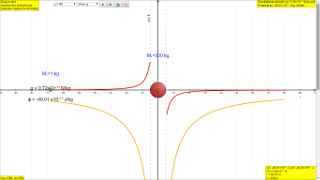gravity06

### 7.3.7 Gravitational Potential (symbol: ϕ and units: J kg-1)

The gravitational potential, ϕ , at a point due to the gravitational field set up by a mass M is defined as the work done per unit mass in bringing a point mass from infinity to that point.

Mathematically, it can be shown that $\varphi =-\genfrac{}{}{0.1ex}{}{GM}{r}$

### 7.3.7.1 Equation U = mϕ

1) This expression $\varphi =-\genfrac{}{}{0.1ex}{}{GM}{r}$ is similar to the expression for gravitational potential energy, $U=-m\genfrac{}{}{0.1ex}{}{GM}{r}$. and they are related by U = mϕ.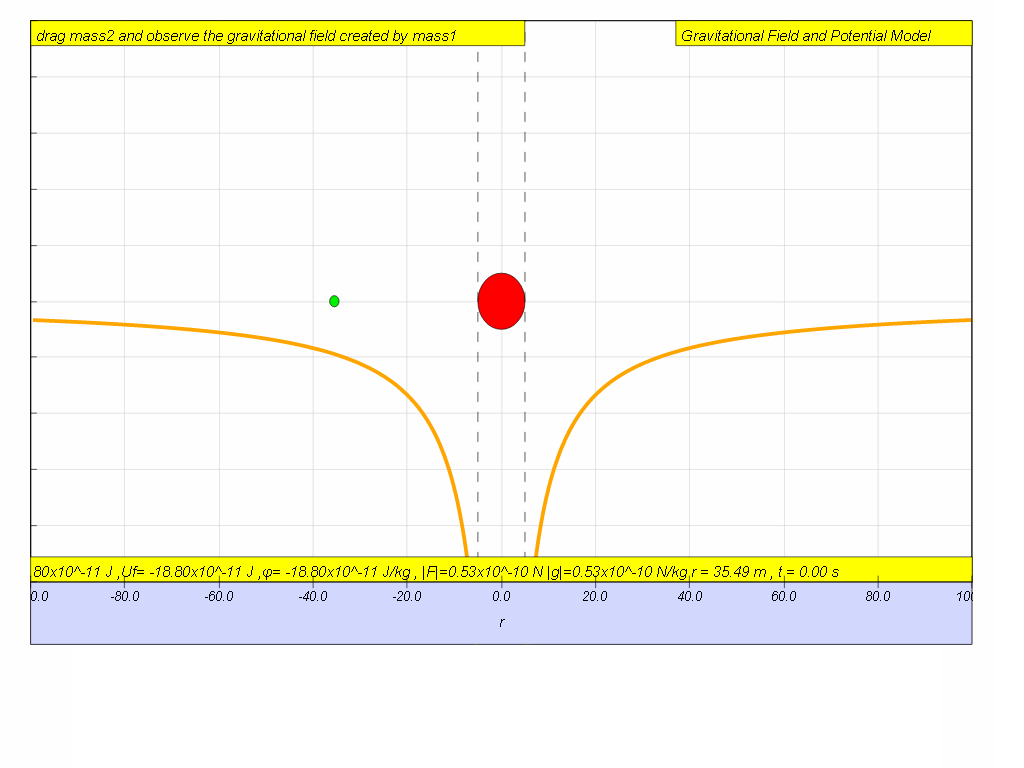### 7.3.7.2 Scalar quantity

2)    Gravitational potential is a scalar quantity. (i.e. it has no direction and a negative value simply means it is less than zero).

### 7.3.7.3 Always negative

3)    This expression implies that ϕ is also always negative (less than zero) and by convention, the gravitational potential at infinity is also taken to be zero (maximum).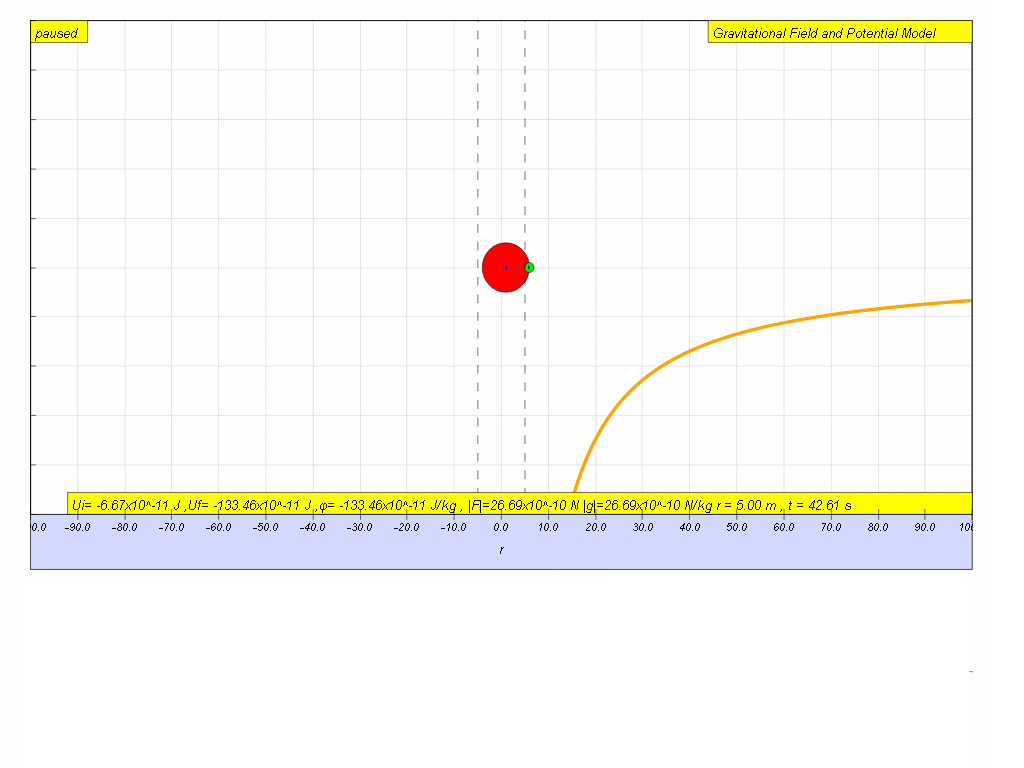### 7.3.7.4 Independent of test mass

4)    Similar to gravitational field strength $g=\genfrac{}{}{0.1ex}{}{GM}{{r}^{2}}$, gravitational potential $\varphi =-\genfrac{}{}{0.1ex}{}{GM}{r}$ is also independent of the mass of the point mass, m.

### 7.3.7.5 Vary according to inverse law with distance r

5)    As distance r of the point mass from source mass increases, ϕ increases according to the equation $\varphi =-\genfrac{}{}{0.1ex}{}{GM}{r}$ .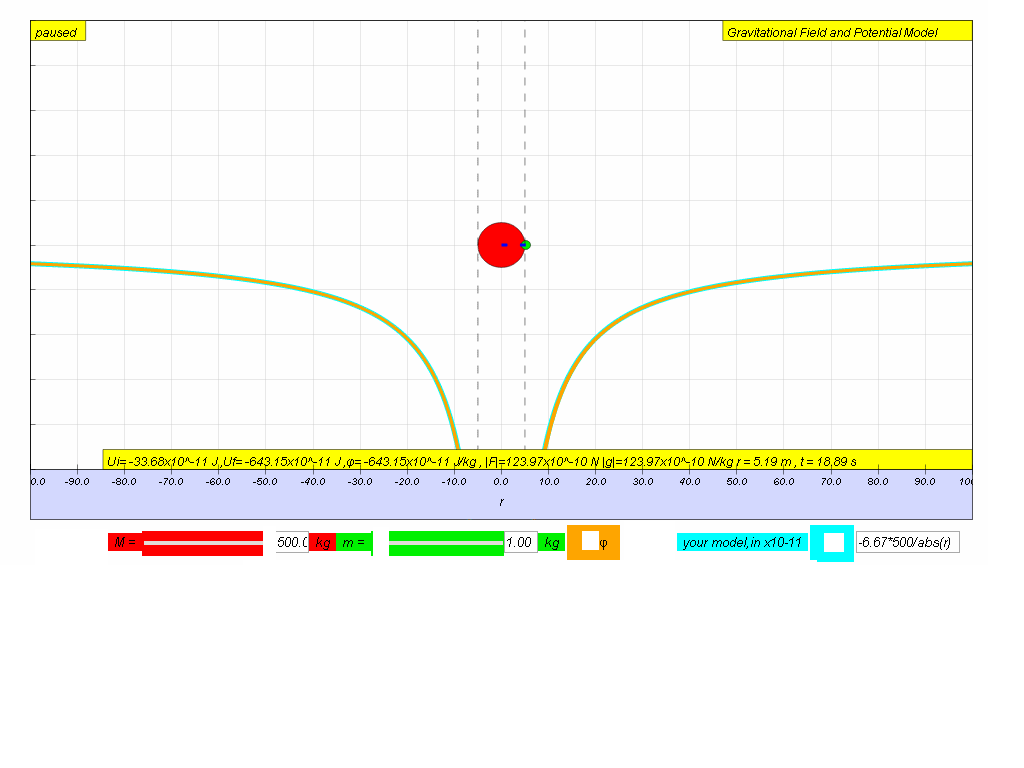### 7.3.8 Activity to do

ICT inquiry worksheet 1 (C) and (D), as well as the "G field and potential" EJS here. The HTML5 version is below, the Java worksheet customization to HTML5 is work in progress.

### 7.3.9 Summary

 symbol g ϕ name Field strength Potential units N kg-1 or m s-2 J kg-1 meaning Gravitational force per unit mass Gravitational potential energy per unit mass quantity vector scalar equation $\mathrm{|g|}=\genfrac{}{}{0.1ex}{}{GM}{{r}^{2}}$ towards the centre of the source mass $\varphi =-\genfrac{}{}{0.1ex}{}{GM}{r}$ relationship to mass Force, $F=\frac{G{M}_{1}{M}_{2}}{{r}^{2}}$ = mg Potential energy, $U=-m\genfrac{}{}{0.1ex}{}{GM}{r}$ = mϕ graph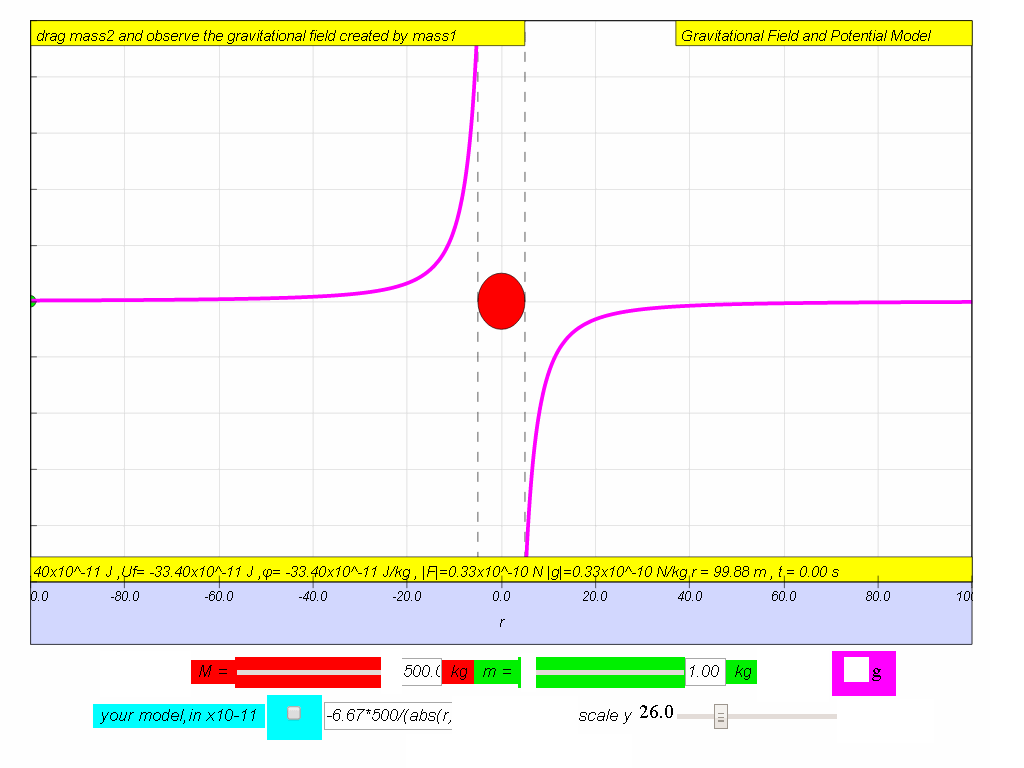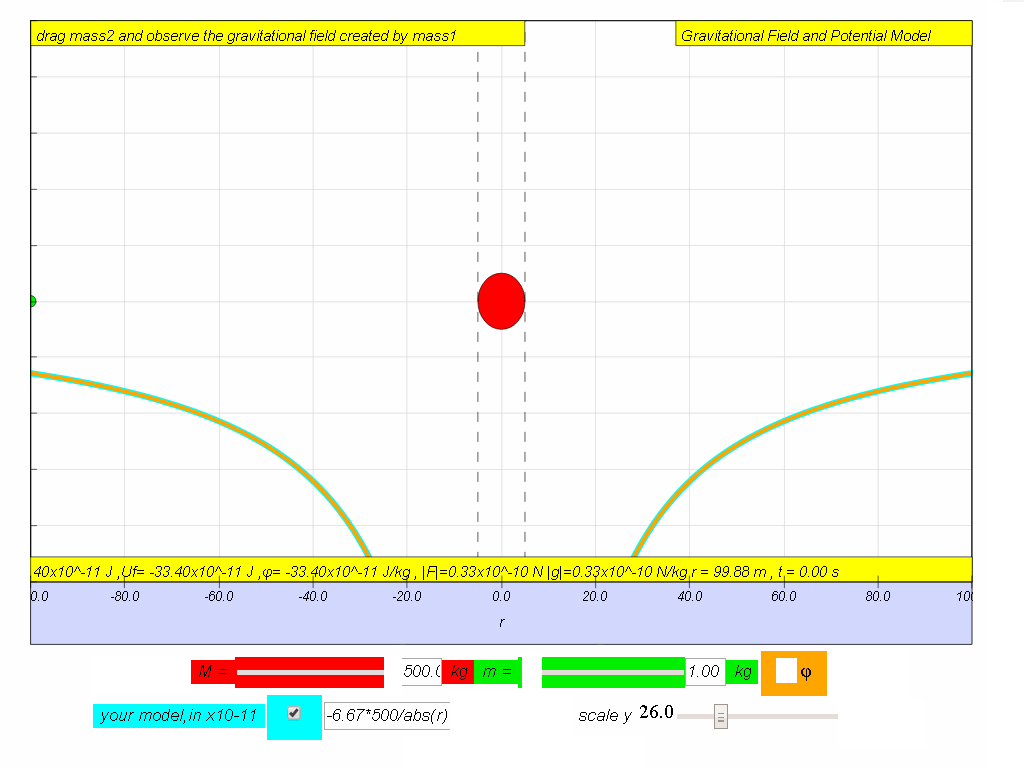computer model if M = 500. -6.67*500/(abs(r)*r) -6.67*500/abs(r)

### 7.3.10 Modeling

Try to input your own model for potential until you achieve a close fit to the data set graph from potential.
Hint:
What is the value of M is the model?
no need to key in x10-11
abs in JavaScript is absolute |   | that always make the value positive.
try something like -6.67*500/abs(r) in the equivalent for $\varphi =-\genfrac{}{}{0.1ex}{}{GM}{r}$

### Translations

Code Language Translator Run### Software Requirements

SoftwareRequirements

 Android iOS Windows MacOS with best with Chrome Chrome Chrome Chrome support full-screen? Yes. Chrome/Opera No. Firefox/ Samsung Internet Not yet Yes Yes cannot work on some mobile browser that don't understand JavaScript such as..... cannot work on Internet Explorer 9 and below

### Credits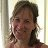This email address is being protected from spambots. You need JavaScript enabled to view it.; Anne Cox; Wolfgang Christian; Francisco Esquembre

### end faq

http://iwant2study.org/lookangejss/02_newtonianmechanics_7gravity/ejss_model_gravity06/gravity06_Simulation.xhtml

## Resources

Rating 5.00 (2 Votes)